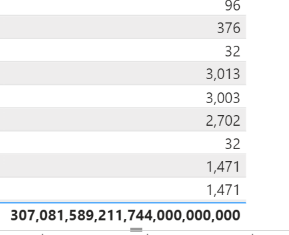cancel
Showing results for
Did you mean:Helper IV

## DAX Measure Total Wrong

Hi All,

I'm struggling to get the  grand total right, its computing fine on row by row basis but the grand total show exponential large amount.

The last reutn statement will check if the row contains unique value and I need to add somehting in there to tell sum the columns after the conversion to currency is done.

```Test USDRR =
VAR gggest = SUMX ( 'Policy (measures)', [Gross] )

VAR AggPremum =

SUMX(
SUMMARIZE (
'Pol (measures)',
'Pol (measures)'[Reporting Currency],
'Pol (measures)'[Policy ID]
),
"@ToUSDRates",
IF (
ISBLANK (
LOOKUPVALUE (
'Exchange Rate'[ExchangeRate Factor],
'Exchange Rate'[Currency From], 'Pol (measures)'[Reporting Currency],
'Exchange Rate'[Currency To], "USD"
)
),
1,
LOOKUPVALUE (
'Exchange Rate'[ExchangeRate Factor],
'Exchange Rate'[Currency From], 'Pol (measures)'[Reporting Currency],
'Exchange Rate'[Currency To], "USD"
)
),
"@AmountinSett", gggest
)
, [@AmountinSett] * [@ToUSDRates]

)

RETURN

IF(HASONEVALUE('Pol (measures)'[Policy ID]), AggPremum, ???? ( How to Get
Total Amount ) ```

6 REPLIES 6Super User

Hi,

I am not sure how your desired visualization looks like, but please try writing two measures like below and check if it suits your requirement.

``````Test USDRR =
VAR gggest =
SUMX ( 'Policy (measures)', [Gross] )
VAR AggPremum =
SUMX (
SUMMARIZE (
'Pol (measures)',
'Pol (measures)'[Reporting Currency],
'Pol (measures)'[Policy ID]
),
"@ToUSDRates",
IF (
ISBLANK (
LOOKUPVALUE (
'Exchange Rate'[ExchangeRate Factor],
'Exchange Rate'[Currency From], 'Pol (measures)'[Reporting Currency],
'Exchange Rate'[Currency To], "USD"
)
),
1,
LOOKUPVALUE (
'Exchange Rate'[ExchangeRate Factor],
'Exchange Rate'[Currency From], 'Pol (measures)'[Reporting Currency],
'Exchange Rate'[Currency To], "USD"
)
),
"@AmountinSett", gggest
),
[@AmountinSett] * [@ToUSDRates]
)
RETURN
AggPremum
``````

``````Test USDRR Total Fix measure =
SUMX ( 'Policy (measures)', [Test USDRR] )
``````

If this post helps, then please consider accepting it as the solution to help other members find it faster, and give a big thumbs up.Helper IV

Hi Jihwan,

``````Test USDRR Total Fix measure =
SUMX ( 'Policy (measures)', [Test USDRR] )``````

but this is causing perfomrace issue , and sometimes prompting me to add less columns values/ filter.``````Test USDRR =
VAR gggest =
SUMX (
'Pol (measures) (measures)',
)
VAR AggPremum =
SUMX(
SUMMARIZE (
'Pol (measures)',
'Pol (measures)'[Reporting Currency],
'Pol (measures)'[Policy ID]
),
"@ToUSDRates",
IF (
ISBLANK (
LOOKUPVALUE (
'Exchange Rate'[ExchangeRate Factor],
'Exchange Rate'[Currency From], 'Pol (measures)'[Reporting Currency],
'Exchange Rate'[Currency To], "USD"
)
),
1,
LOOKUPVALUE (
'Exchange Rate'[ExchangeRate Factor],
'Exchange Rate'[Currency From], 'Pol (measures)'[Reporting Currency],
'Exchange Rate'[Currency To], "USD"
)
),
"@AmountinSett", gggest
),
IF (
HASONEFILTER ( 'Pol (measures)' [Policy ID]  ),
[@AmountinSett] * [@ToUSDRates],
SUMX (
FILTER (
'Pol (measures)',
[@AmountinSett] > 10000000
),
[@AmountinSett] * [@ToUSDRates]
)
)
)
RETURN
AggPremum``````Super User

Hi,

I am not sure how your Policy(measures) table looks like, but please try writing DAX measure something like below. Or, please share your sample pbix file's link, and then I can try to look into it to come up with a more accurate solution.

``````Test USDRR Total Fix measure =
SUMX (
DISTINCT ( 'Policy (measures)'[Your desired column that is in the visualization] ),
[Test USDRR]
)
``````

If this post helps, then please consider accepting it as the solution to help other members find it faster, and give a big thumbs up.Helper IVSuper User

@EricShahi First, please vote for this idea: https://ideas.powerbi.com/ideas/idea/?ideaid=082203f1-594f-4ba7-ac87-bb91096c742e

This looks like a measure totals problem. Very common. See my post about it here: https://community.powerbi.com/t5/DAX-Commands-and-Tips/Dealing-with-Measure-Totals/td-p/63376

Also, this Quick Measure, Measure Totals, The Final Word should get you what you need:
https://community.powerbi.com/t5/Quick-Measures-Gallery/Measure-Totals-The-Final-Word/m-p/547907

Also: https://youtu.be/uXRriTN0cfY
And: https://youtu.be/n4TYhF2ARe8

Become an expert!: Enterprise DNA
External Tools: MSHGQM
Latest book!:
Mastering Power BI 2nd EditionDAX is easy, CALCULATE makes DAX hard...Helper IV

Hi Greg,

I read thorugh your documnets around the issue on the measures totals, I tried to used your techinique but still no luck.

Test USDRR =
VAR gggest =
SUMX (
'Pol (measures) (measures)',
)
VAR AggPremum =
SUMX(
SUMMARIZE (
'Pol (measures)',
'Pol (measures)'[Reporting Currency],
'Pol (measures)'[Policy ID]
),
"@ToUSDRates",
IF (
ISBLANK (
LOOKUPVALUE (
'Exchange Rate'[ExchangeRate Factor],
'Exchange Rate'[Currency From], 'Pol (measures)'[Reporting Currency],
'Exchange Rate'[Currency To], "USD"
)
),
1,
LOOKUPVALUE (
'Exchange Rate'[ExchangeRate Factor],
'Exchange Rate'[Currency From], 'Pol (measures)'[Reporting Currency],
'Exchange Rate'[Currency To], "USD"
)
),
"@AmountinSett", gggest
),
IF (
HASONEFILTER ( 'Pol (measures)' [Policy ID]  ),
[@AmountinSett] * [@ToUSDRates],
SUMX (
FILTER (
'Pol (measures)',
[@AmountinSett] > 10000000
),
[@AmountinSett] * [@ToUSDRates]
)
)
)
RETURN
AggPremum